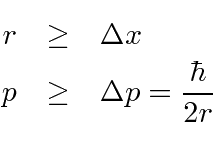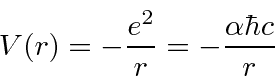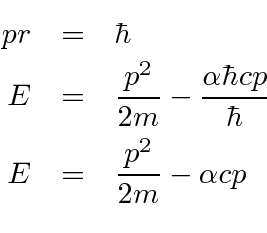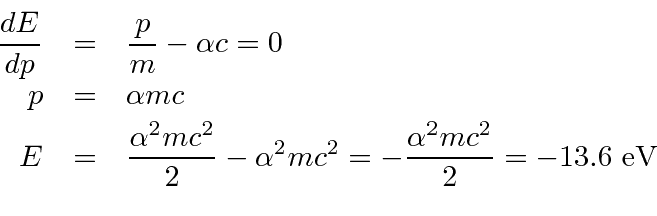### Estimate the Hydrogen Ground State Energy

The reason the Hydrogen atom (and other atoms) is so large is the essentially uncertainty principle. If the electron were confined to a smaller volume,would increase, causingto increase on average. The energy would increase not decrease.

We can use the uncertainty principle to estimate the minimum energy for Hydrogen. This is not a perfect calculation but it is more correct than the Bohr model. The idea is that the radius must be larger than the spread in position, and the momentum must be larger than the spread in momentum.This is our formula for the potential energy in terms of the dimensionless fine structure constant.Lets estimate the energyand put in the effect of the uncertainty principle.Differentiate with respect toand set equal to zero to get the minimum.Note that the potential energy is just (-2) times the kinetic energy (as we expect from the Virial Theorem). The ground state energy formula is correct.

We can also estimate the radius.The ground state of Hydrogen has zero (orbital) angular momentum. It is not moving in a circular orbit as Bohr hypothesized. The electron just has a probability distribution that is spread out over about 1 Å. If it were not spread out, the energy would go up.

Jim Branson 2013-04-22